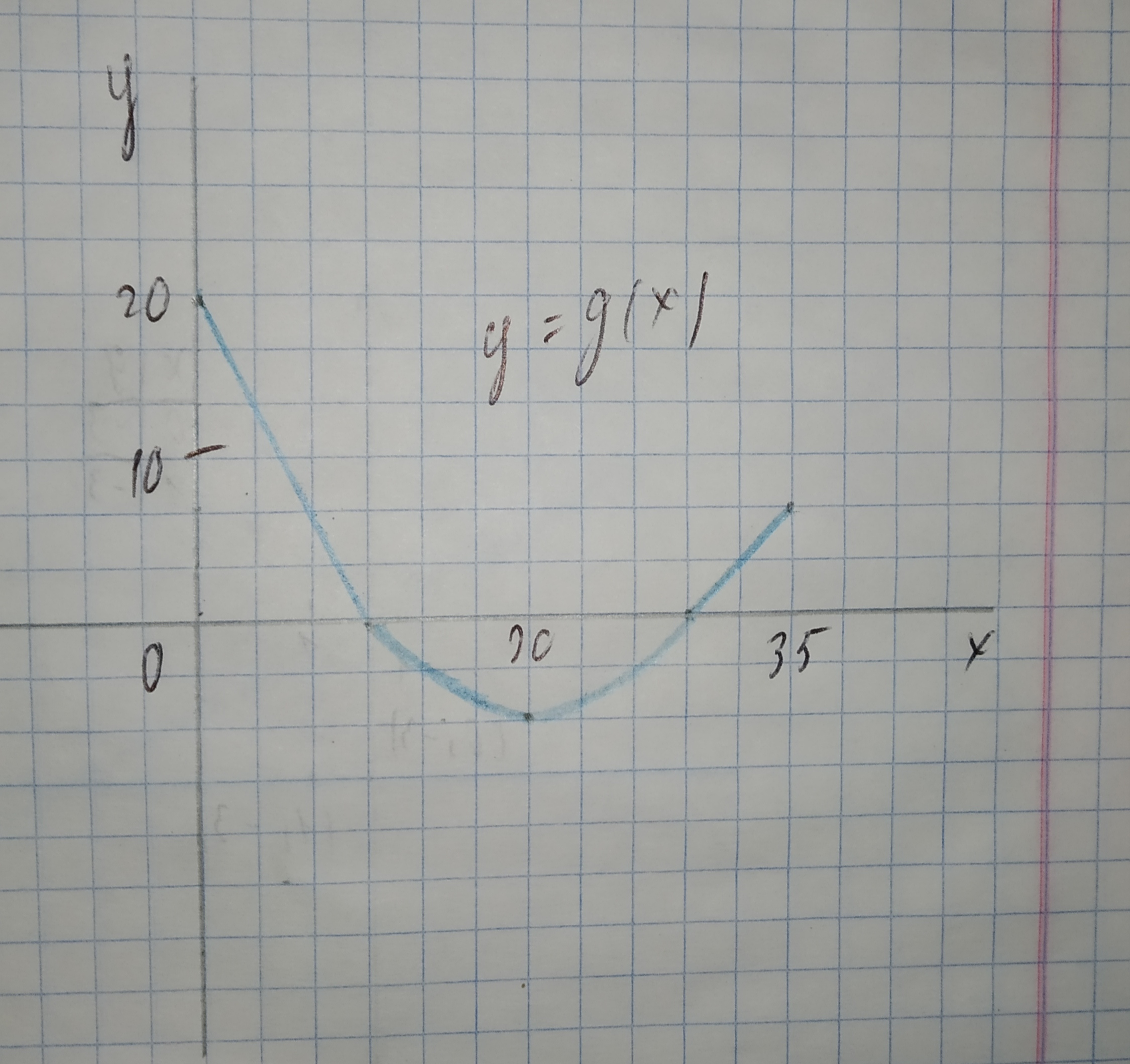#### Didn’t find what you are looking for?Applications of integrals### The graph of g consists of two straight lines and a semicircle. Use it to eveluate the integral.$$\displaystyle{\int_{{0}}^{{35}}}{g{{\left({x}\right)}}}{\left.{d}{x}\right.}$$

Applications of integrals### The graph of g consists of two straight lines and a semicircle. Use it to eveluate the integral.$$\displaystyle{\int_{{10}}^{{30}}}{g{{\left({x}\right)}}}{\left.{d}{x}\right.}$$

Applications of integrals### The graph of g consists of two straight lines and a semicircle. Use it to eveluate the integral.$$\displaystyle{\int_{{0}}^{{10}}}{g{{\left({x}\right)}}}{\left.{d}{x}\right.}$$